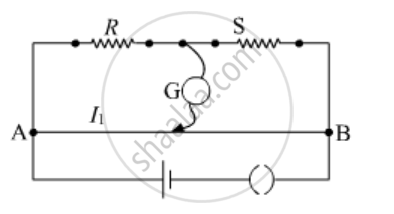Department of Pre-University Education, KarnatakaPUC Karnataka Science Class 12

# In a Metre Bridge, the Balance Point is Found at a Distance L1 With Resistances R and S as Shown in the Figure.An Unknown Resistance X is Now Connected in Parallel to the Resistance S and the Balance Point is Found at a Distance L2. Obtain a Formula for X in Terms of L1, L2 and S. - Physics

In a metre bridge, the balance point is found at a distance l1 with resistances R and S as shown in the figure.An unknown resistance X is now connected in parallel to the resistance S and the balance point is found at a distance l2. Obtain a formula for X in terms of l1, l2 and S.#### Solution

When resistance R and S are connected :
Since balance point is found at a distance l1 from the zero end,
l1 and,

(100 − l1 )

therefore R/S=l_1/(100-l_1).............(i)

When unknown resistance X is connected in parallel to S , then the effective resistance in the right gap, S'=(SX)/(S+X) ...............(ii)
Now, the balance point is obtained at a distance l2 from the zero end,
R ∝ l2
S' ∝ (100−l2)

therefore R/S'=l_2/(100-l_2).............(iii)

Substituting the value of S' from (ii),

(R(S+X))/(SX)=l_2/(100-l_2)............(iv)

Dividing equation (iv) by (i),

(S+X)/X=l_2/(100-l_2)xx(100-l_1)/l_1

S/X+1=(l_2(100-l_1))/(l_1(100-l_2))

S/X=(l_2(100-l_1))/(l_1(100-l_2))-1

S/X=(100l_2-l_1l_2-100l_1+l_1l_2)/(l_1(100-l_2))

S/X=(100(l_2-l_1))/(l_1(100-l_2))

X=(l_1(100-l_2))/(100(l_2-l_1)) S

Concept: Metre Bridge
Is there an error in this question or solution?